Jane is fond of playing guitar. So she keeps a guitar beside, or adjacent to, her bed.Many of us are fond of books and like to place books and other favorite stuff adjacent to our bed.

Entering your classroom, you might always want your best friend to sit just next to you or on a desk adjacent to yours.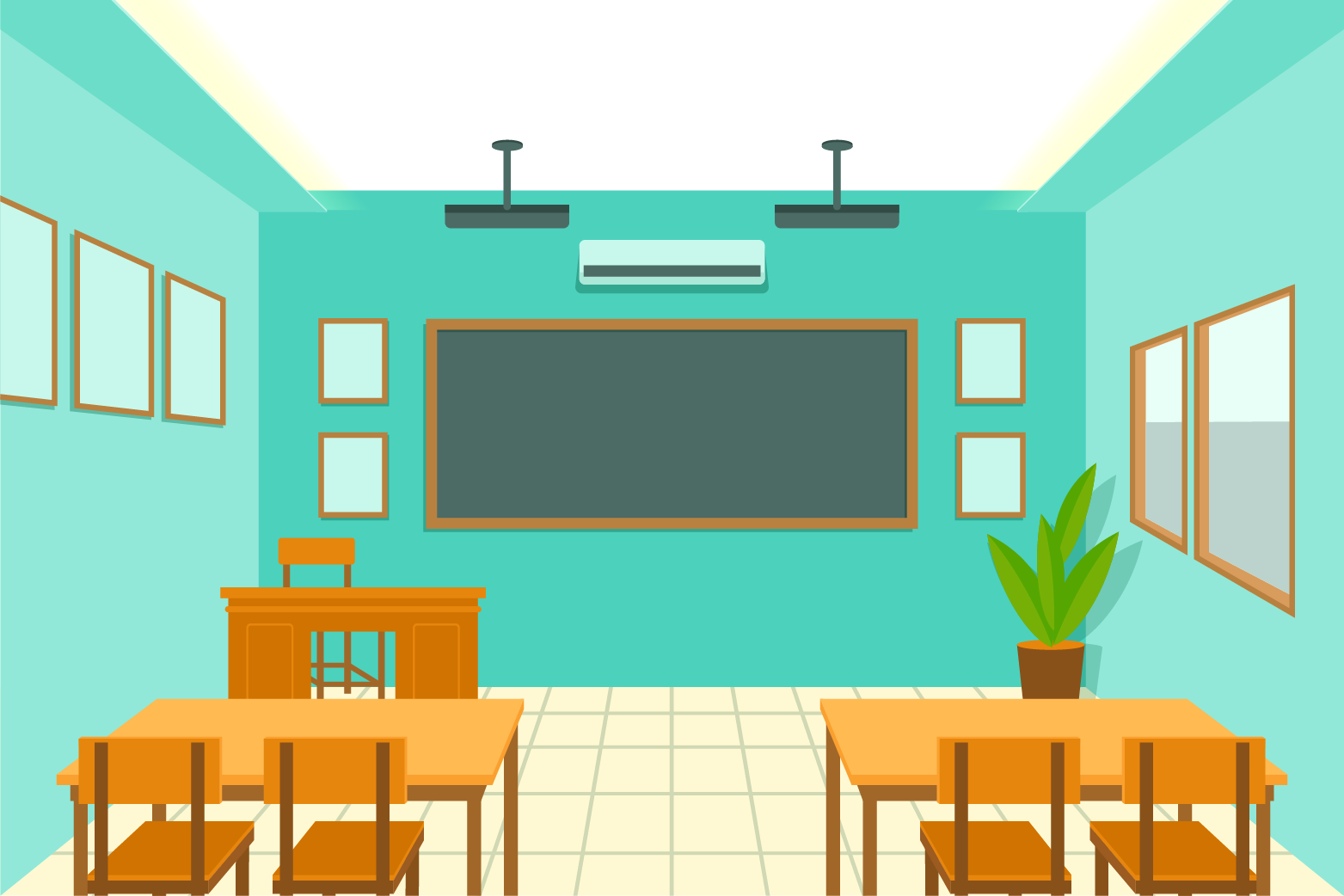The word "adjacent" comes from Latin adjacere, that is, "to lie near." The prefix "ad" means "to" and "jacere" means "to lie, throw."

The word "adjacent" is often followed by the preposition "to." For example, "My sister's room is adjacent to mine."

Adjacent angles can then be considered as those which share a common side.

Let's have a look!

Check out the interesting examples to know more about this lesson and try your hand at solving a few interactive questions at the end of the page.

## Lesson Plan

The meaning of adjacent is nearby or next to.

An example of adjacent is two buildings that are next to one another.

We usually consider the people in/around our street to be our neighbors.

Who's our next-door neighbor then?

The person who lives in the house or apartment adjacent to ours.Adjacent can refer to two things, touching each other or sharing the same wall or border.

What about the two lines that meet at a polygon vertex?

Can they be considered as adjacent sides?

In triangles and other polygons, two of the sides that meet at the vertex of a polygon are known as adjacent sides.

In the case of a right triangle,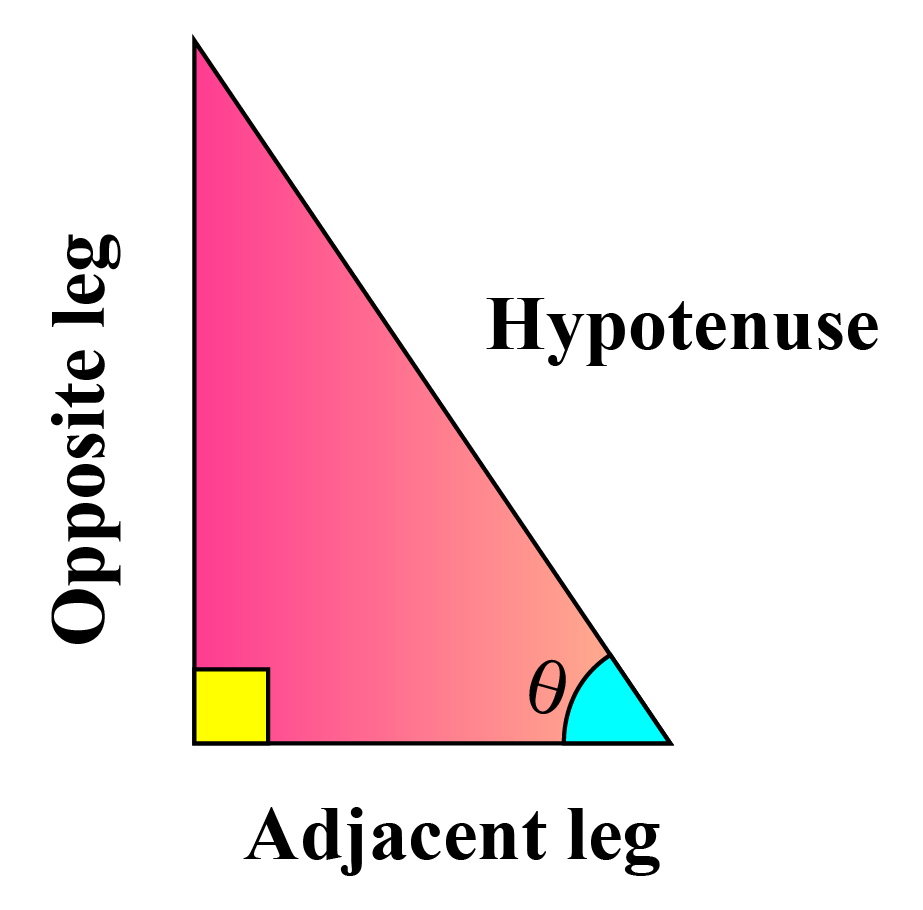• the hypotenuse is the longest side.
• an "opposite" side is the one across from a given angle.
• an "adjacent" side is next to the given angle.

What about the sides of rectangles and squares?

## Where Can We Use the Concept Of Adjacent?

In math, adjacent can be many things, but the concept of adjacent is applied mainly to line segments and angles.

Any two angles that share

• a common ray or side
• a common vertex
• and whose interiors do not overlap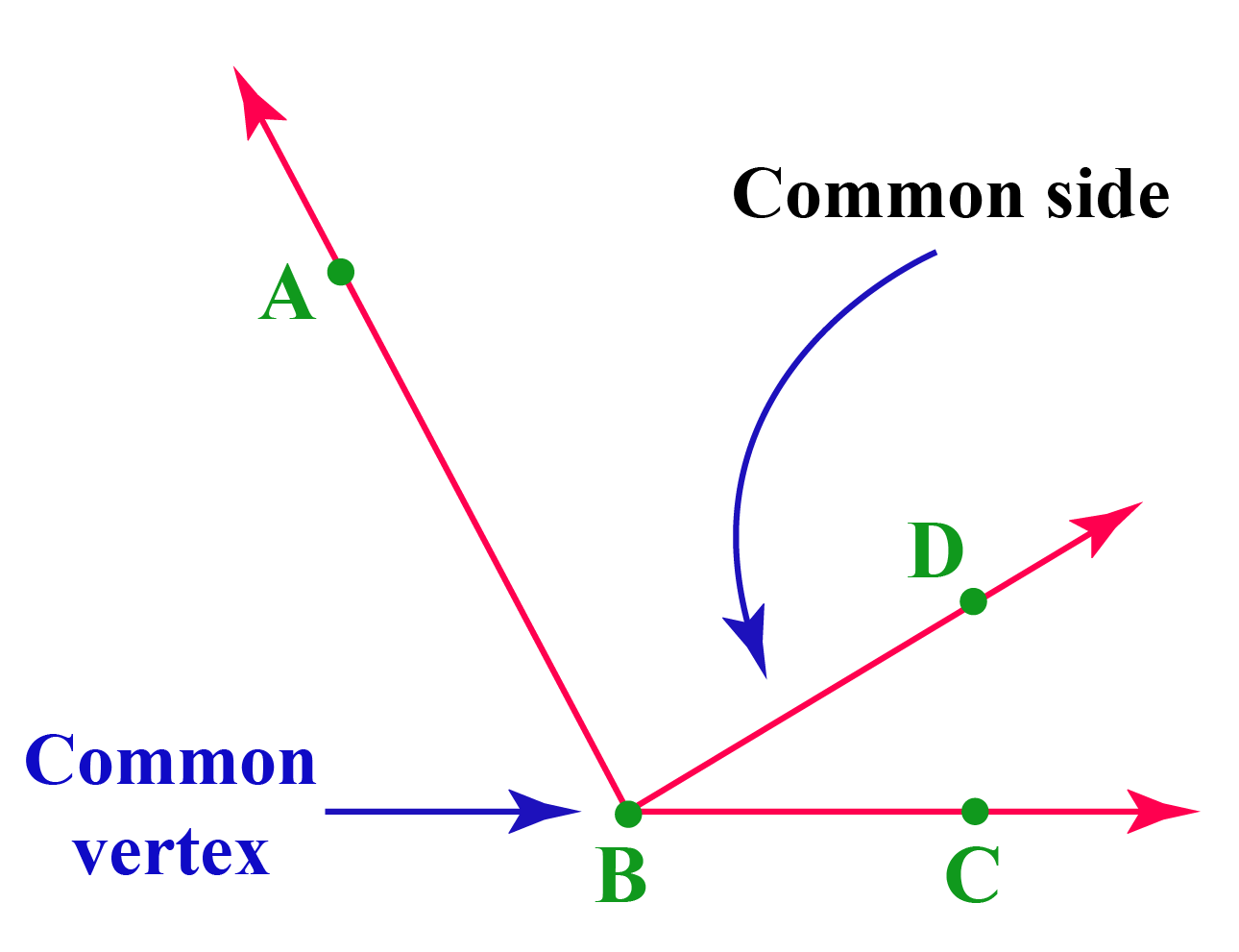Interiors of $$\angle ABD$$ and $$\angle CBD$$ don’t overlap, and hence they are adjacent angles.

Look at the following figures.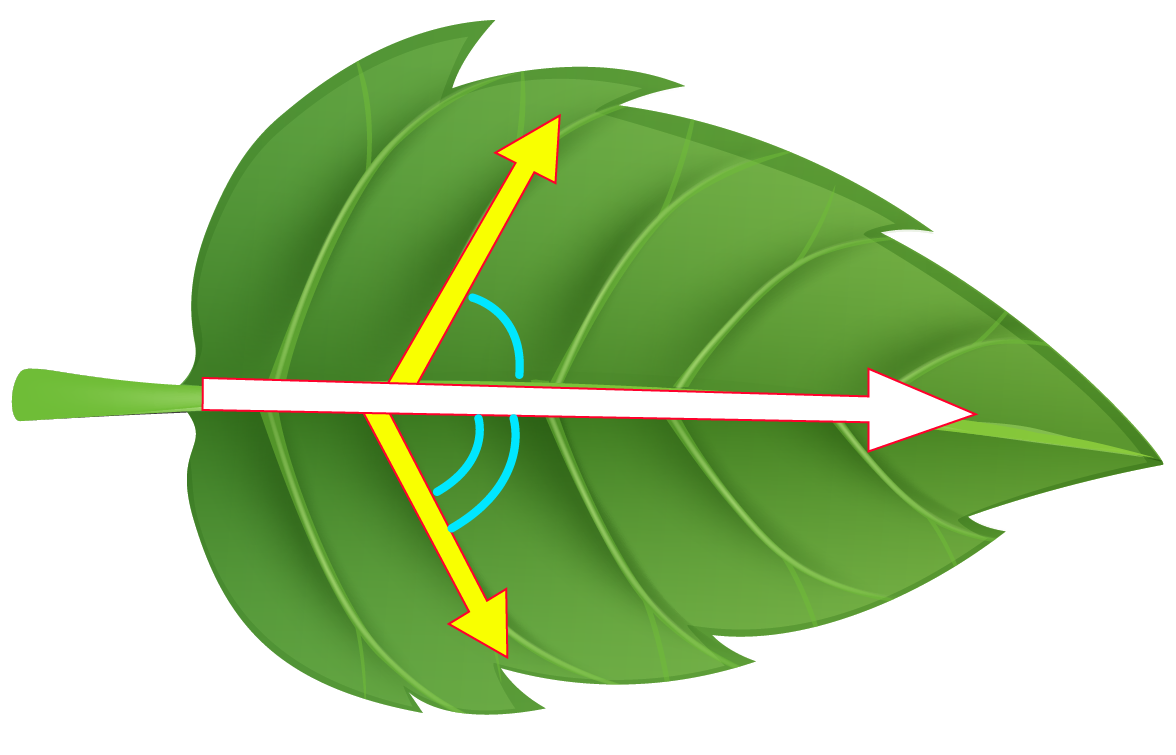The pattern formed by the veins of a leaf actually form adjacent angle pairs.

##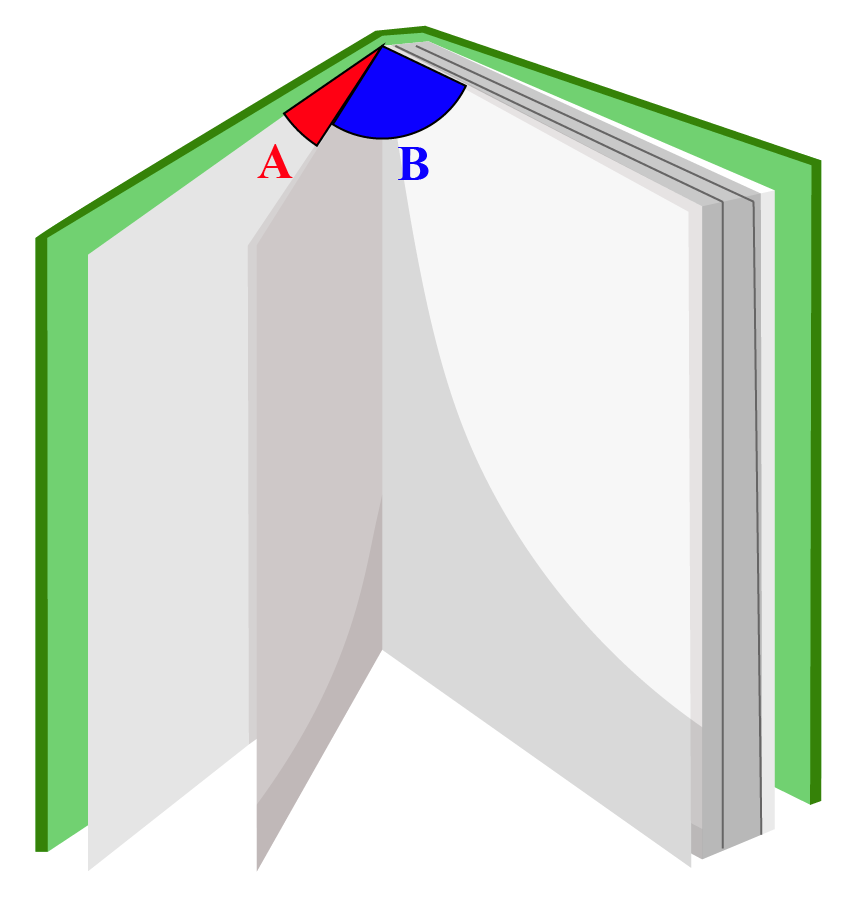When you open a book, it looks like this.

In A and B, there are angles that are placed next to each other.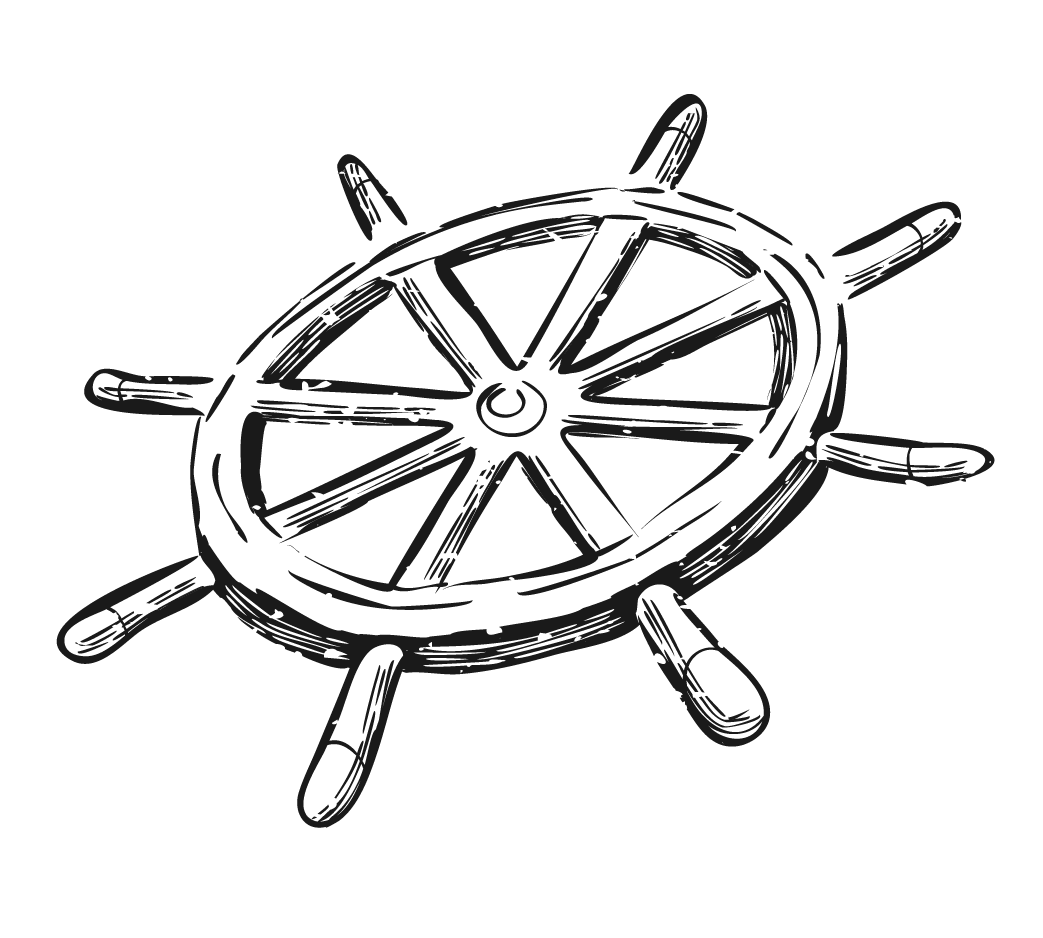At the center of the wheel, there are 8 angles being formed, lying next to one another.

So, adjacent angles have a common arm and a common vertex but no common interior points.

## Solved Examples

 Example 1

Look at the hands of the clock!

Do they form a pair of adjacent angles?Solution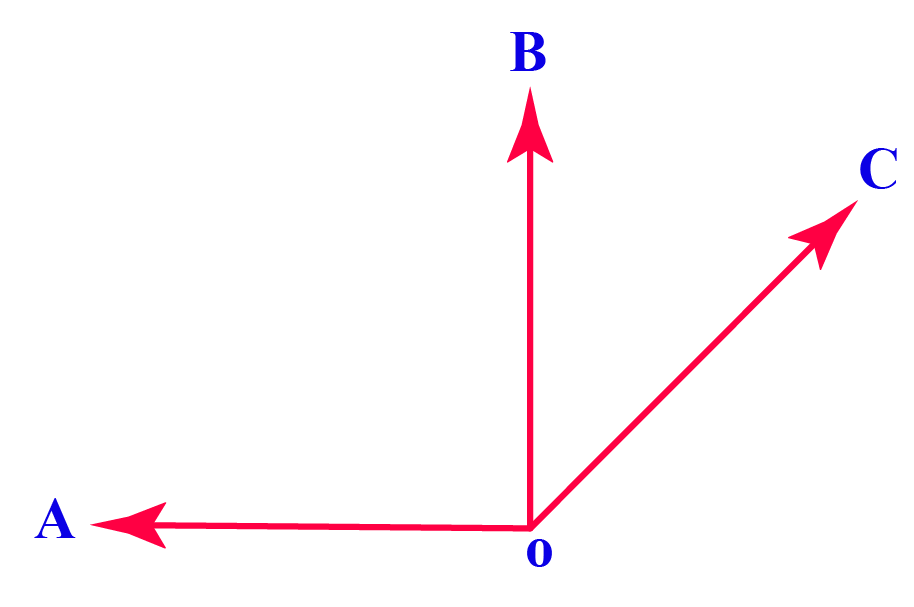The hour hand forms another angle with the second hand represented as∠AOB.

The minute hand and the second hand of the clock form one angle represented as ∠BOC.

Both these pair of angles, i.e., ∠AOB and ∠BOC lie next to each other and form a pair of adjacent angles.

 ∠AOB and ∠BOC form adjacent angle pairs.
 Example 2

Are the angles marked as 1 and 2 in the following figures adjacent? Give reasons for your answers.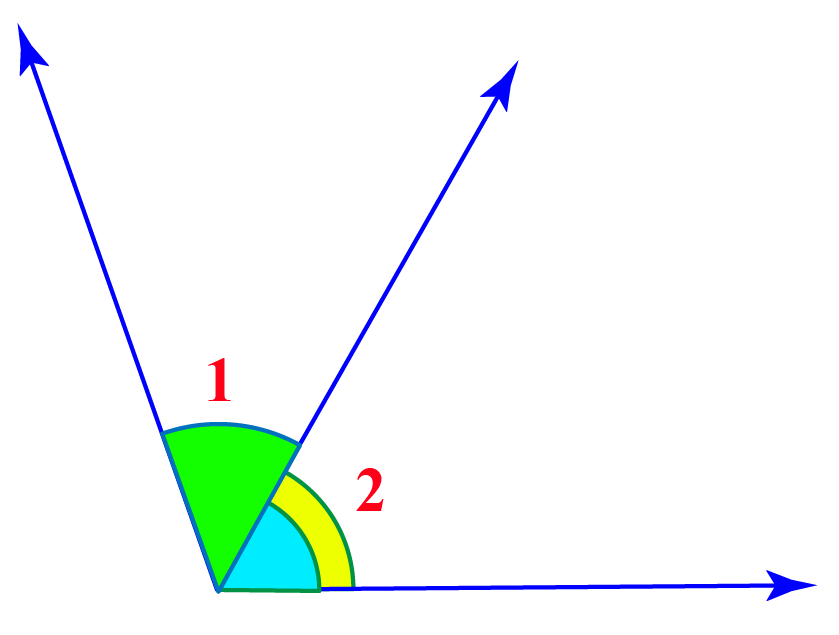Solution

Let’s label the rays in the figure as follows.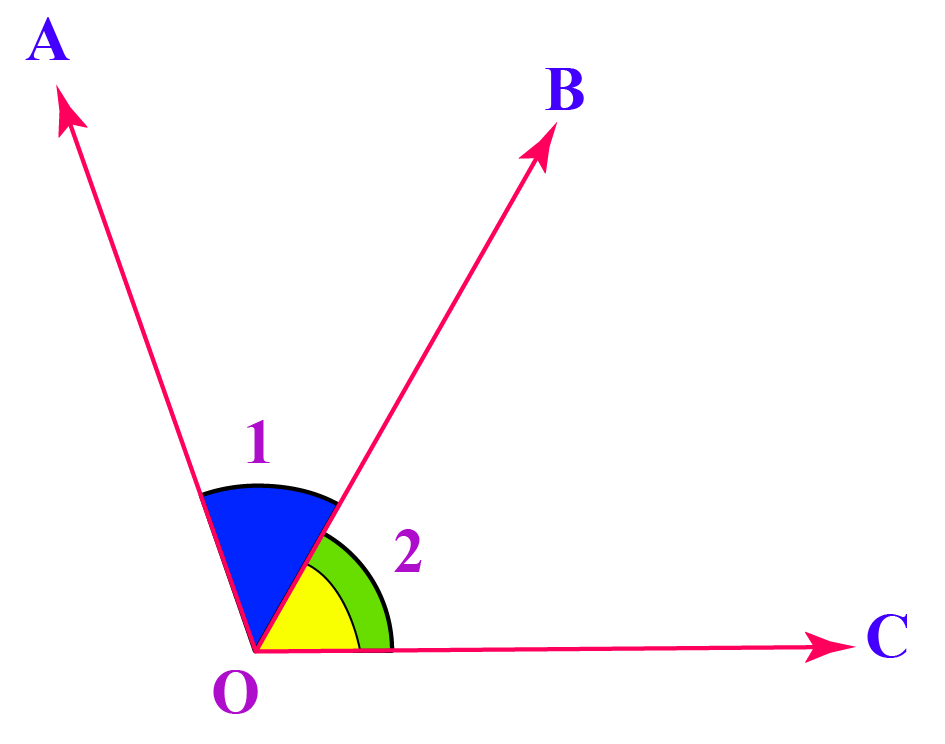Clearly, $$\angle 1$$ and $$\angle 2$$ have a common vertex O and a common arm OB.

They have non-common arms OA  and OC on both sides of the common arm OB.

So, $$\angle 1$$ and $$\angle 2$$ are adjacent angles.

 Yes, $$\angle 1$$ and $$\angle 2$$ are adjacent angles.
 Example 3

List 5 pairs of adjacent angles in the following figure.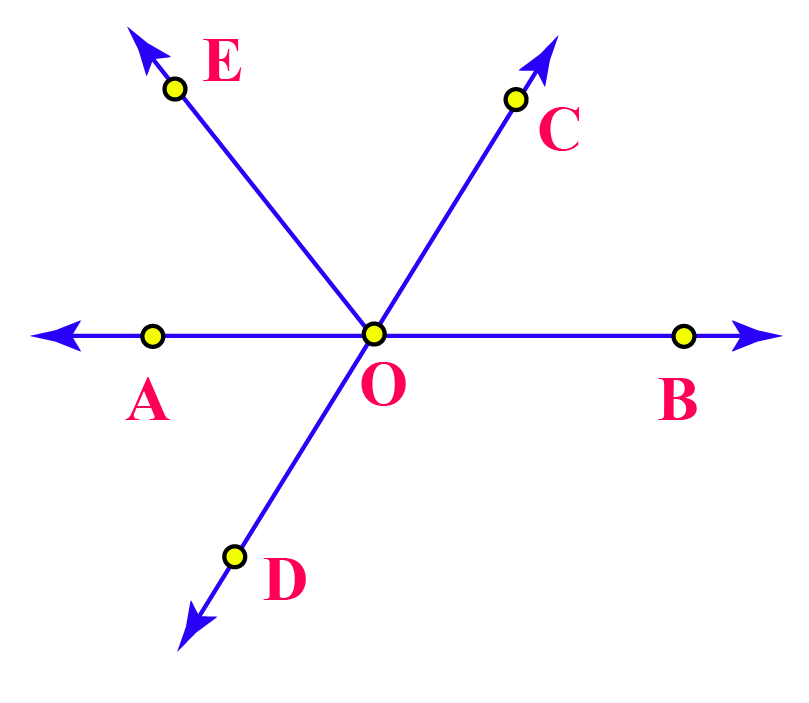Solution

Five pairs of adjacent angles are given below.

1. $$\angle AOE, \angle EOC$$
2. $$\angle EOC, \angle COB$$
3. $$\angle AOC, \angle COB$$
4. $$\angle COB, \angle BOD$$
5. $$\angle EOB, \angle BOD$$Think Tank
1. Can two obtuse  angles form a pair of adjacent angles?
2. Can an acute angle be adjacent to an obtuse angle?
3. What about the step inclining the divider or cross streets? Can these be considered as examples of adjacent?

## Interactive Questions

Try these Questions based on the Number Line in the worksheet given below.

## Let's Summarize

At Cuemath, our team of math experts is dedicated to making learning fun for our favorite readers, the students!

Through an interactive and engaging learning-teaching-learning approach, the teachers explore all angles of a topic.

Be it worksheets, online classes, doubt sessions, or any other form of relation, it’s the logical thinking and smart learning approach that we at Cuemath believe in.

## 1. What is the definition of adjacent?

Two things are adjacent when they touch each other or share the same wall or border.

## 2. What is the definition of adjacent angles?

Any two angles that share

• a common ray or side
• a common vertex
• and whose interiors do not overlap